Methods and formulas for Simple Regression

Select the method or formula of your choice.

Adj MS – Error

The Mean Square of the error (also abbreviated as MS Error or MSE, and denoted as s2) is the variance around the fitted regression line. The formula is:

Notation

TermDescription
yiith observed response value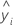ith fitted response
nnumber of observations
pnumber of coefficients in the model, not counting the constant

Adj MS – Regression

The formula for the Mean Square (MS) of the regression is:

Notation

TermDescriptionmean responseith fitted response
pnumber of terms in the model

Adj MS – Total

The formula for the total Mean Square (MS) is:

Notation

TermDescriptionmean response
yiith observed response value
nnumber of observations

The sum of the squared distances. SS Regression is the portion of the variation explained by the model. SS Error is the portion not explained by the model and is attributed to error. SS Total is the total variation in the data.

SS Regression:
SS Error:
SS Total:

Notation

TermDescription
yi i th observed response value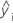i th fitted responsemean response

Coefficient (Coef)

The formula for the coefficient or slope in simple linear regression is:

The formula for the intercept (b0) is:

In matrix terms, the formula that calculates the vector of coefficients in multiple regression is:

b = (X'X)-1X'y

Notation

TermDescription
yiith observed response valuemean response
xiith predictor value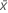mean predictor
Xdesign matrix
yresponse matrix

Degrees of freedom (DF)

The degrees of freedom for each component of the model are:

Sources of variation DF
Regression p
Error n – p – 1
Total n – 1

If your data meet certain criteria and the model includes at least one continuous predictor or more than one categorical predictor, then Minitab uses some degrees of freedom for the lack-of-fit test. The criteria are as follows:
• The data contain multiple observations with the same predictor values.
• The data contain the correct points to estimate additional terms that are not in the model.

Notation

TermDescription
n number of observations
p number of coefficients in the model, not counting the constant

Fit

Notation

TermDescription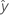fitted value
xkkth term. Each term can be a single predictor, a polynomial term, or an interaction term.
bkestimate of kth regression coefficient

F-value

The formulas for the F-statistics are as follows:

F(Regression)
F(Term)
F(Lack-of-fit)

Notation

TermDescription
MS RegressionA measure of the variation in the response that the current model explains.
MS ErrorA measure of the variation that the model does not explain.
MS TermA measure of the amount of variation that a term explains after accounting for the other terms in the model.
MS Lack-of-fitA measure of variation in the response that could be modeled by adding more terms to the model.
MS Pure errorA measure of the variation in replicated response data.

P-value – Coefficients table

The two-sided p-value for the null hypothesis that a regression coefficient equals 0 is:

The degrees of freedom are the degrees of freedom for error, as follows:

np – 1

Notation

TermDescription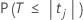The cumulative distribution function of the t distribution with degrees of freedom equal to the degrees of freedom for error.
tjThe t statistic for the jth coefficient.
nThe number of observations in the data set.
pThe sum of the degrees of freedom for the terms. The terms do not include the constant.

P-value – Analysis of variance table

This p-value is for the test of the null hypothesis that all of the coefficients that are in the model equal zero, except for the constant coefficient. The p-value is a probability that is calculated from an F-distribution with the degrees of freedom (DF) as follows:

Numerator DF
sum of the degrees of freedom for the term or the terms in the test
Denominator DF
degrees of freedom for error

1 − P(Ffj)

Notation

TermDescription
P(Ffj)cumulative distribution function for the F-distribution
fjf-statistic for the test

Regression equation

For a model with multiple predictors, the equation is:

y = β0 + β1x1 + … + βkxk + ε

The fitted equation is:

In simple linear regression, which includes only one predictor, the model is:

y=ß0+ ß1x1+ε

Using regression estimates b0 for ß0, and b1 for ß1, the fitted equation is:

Notation

TermDescription
yresponse
xkkth term. Each term can be a single predictor, a polynomial term, or an interaction term.
ßkkth population regression coefficient
εerror term that follows a normal distribution with a mean of 0
bkestimate of kth population regression coefficientfitted response

Residual (Resid)

Notation

TermDescription
eii th residual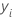i th observed response value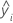i th fitted response

R-sq

R2 is also known as the coefficient of determination.

Notation

TermDescription
yi i th observed response valuemean responsei th fitted response

While the calculations for adjusted R2 can produce negative values, Minitab displays zero for these cases.

Notation

TermDescription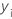ith observed response valueith fitted responsemean response
nnumber of observations
pnumber of terms in the model

R-sq (pred)

While the calculations for R2(pred) can produce negative values, Minitab displays zero for these cases.

Notation

TermDescription
yi i th observed response value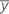mean response
n number of observations
ei i th residual
hi i th diagonal element of X(X'X)–1X'
X design matrix

S

Notation

TermDescription
MSEmean square error

Standard error of the coefficient (SE Coef)

For simple linear regression, the standard error of the coefficient is:

The standard errors of the coefficients for multiple regression are the square roots of the diagonal elements of this matrix:

Notation

TermDescription
xiith predictor valuemean of the predictor
Xdesign matrix
X'transpose of the design matrix
s2mean square error

Standardized residual (Std Resid)

Standardized residuals are also called "internally Studentized residuals."

Notation

TermDescription
ei i th residual
hi i th diagonal element of X(X'X)–1X'
s2 mean square error
Xdesign matrix
X'transpose of the design matrix

T-value

Notation

TermDescription
tjtest statistic for the jth coefficient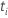jth estimated coefficientstandard error of the jth estimated coefficient

Variance inflation factor (VIF)

Minitab calculates the VIF by regressing each predictor on the remaining predictors and noting the R2value.

Formula

For predictor xj, the VIF is:

Notation

TermDescription
R2( xj)coefficient of determination with xj as the response variable and the other terms in the model as the predictors
By using this site you agree to the use of cookies for analytics and personalized content.  Read our policy